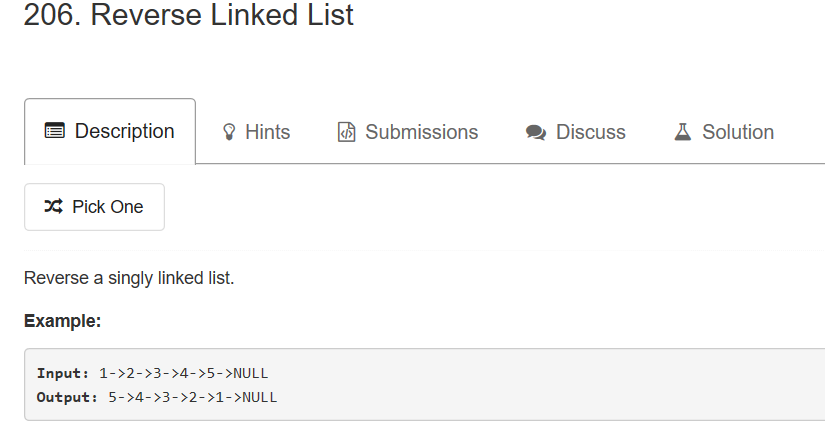5个回答

`````` #include "stdio.h"

struct ListNode {
int val;
struct ListNode *next;
};

ListNode* newh = NULL;
while(p)
{
ListNode* tmp = p -> next;
p -> next = newh;
newh = p;
p = tmp;
}
return newh;
}

{
}
printf("\n");
}

int _tmain(int argc, _TCHAR* argv[])
{
ListNode p5; p5.val = 5; p5.next = NULL;
ListNode p4; p4.val = 4; p4.next = &p5;
ListNode p3; p3.val = 3; p3.next = &p4;
ListNode p2; p2.val = 2; p2.next = &p3;
ListNode p1; p1.val = 1; p1.next = &p2;
PrintList(&p1);
ListNode* newlist = ReverseList(&p1);
PrintList(newlist);
return 0;
}
``````

newh是 1->NULL

newh是 2->1->NULL

newh是 3->2->1->NULL

newh是 4->3->2->1->NULL

newh是 5->4->3->2->1->NULL

`````` #include <iostream>
#include <conio.h>
using namespace std;
template <class T>
{
public:
T data;
{
data=el;
prev=ptr;
next=pt;
};
};
template <class T>
{
private:

public:
{
}
Clear();}
void Clear()
{
while (top != rear)
{
top=top->prev;
delete tmp;

}

}
bool Push(const T item)
{

top->next=tmp;
top=tmp;
return true;

}
bool Pop(T & item)
{
if (top==rear)
{
return false;

}
item = top->data;
tmp=rear->next;
delete rear;
rear =tmp;
return true;
}
void print()
{
while(tmp!=rear)
{
cout<<tmp->data<<"  ";
tmp=tmp->prev;
}
cout<<endl;
}
};
int main()
{int n,i,c;
cout<<"输入n"<<endl;
cin>>n;
int aa;
for(i=0;i<n;i++)
{
cin>>c;
if(c>=0 && c<10)
a[c].Push(c);
else
{
cout<<"第"<<i+1<<"次输入错误,算作无效输入"<<endl;
i--;
}
}

for(i=9;i>=0;i--)
{a[i].print();
while(a[i].Pop(c))
b.Push(c);
}
b.print();
getch();
}

``````alaboboy 回复caozhy: 时间空间是肯定要打折扣的，不过相对方便管理一点，实质是简化而不是繁琐，要不就没有学习堆栈的必要了